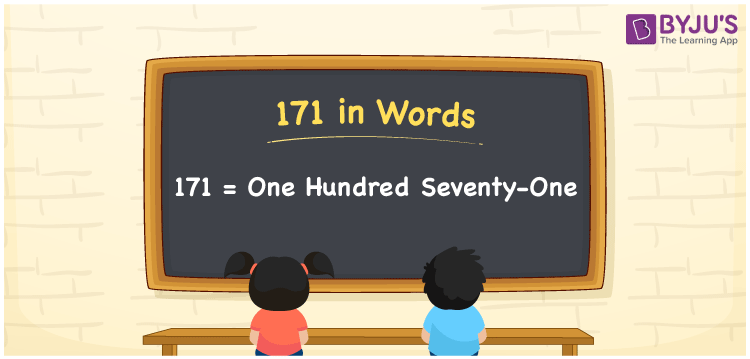# 171 in words

171 in words is written as One Hundred and Seventy One. 171 represents the count or value. The article on Counting Numbers can give you an idea about count or counting. The number 171 is a 3 digit number that is used in expressions related to money, days, distance, length, weight and so on. Let us consider an example for 171. “The door number of that house is One Hundred and Seventy One.”

 171 in words One Hundred and Seventy One One Hundred and Seventy One in Numbers 171

## 171 in English Words## How to Write 171 in Words?

We can convert 171 to words using a place value chart. The number 171 has 3 digits, so let’s make a chart that shows the place value up to 3 digits.

 Hundreds Tens Ones 1 7 1

Thus, we can write the expanded form as:

1 × Hundred + 7 × Ten + 1 × One

= 1 × 100 + 7 × 10 + 1 × 1

= 171

= One Hundred and Seventy One.

171 is the natural number that is succeeded by 170 and preceded by 172.

171 in words – One Hundred and Seventy One.

Is 171 an odd number? – Yes.

Is 171 an even number? – No.

Is 171 a perfect square number? – No.

Is 171 a perfect cube number? – No.

Is 171 a prime number? – No.

Is 171 a composite number? – Yes.

## Solved Example

1. Write the number 171 in expanded form

Solution: 1 × 100 + 7 × 10 + 1 × 1

We can write 171 = 100 + 70 + 1

= 1 × 100 + 7 × 10 + 1 × 1.

## Frequently Asked Questions on 171 in words

Q1

### How to write the number 171 in words?

171 in words is written as One Hundred and Seventy One.
Q2

### Is 171 divisible by 3?

Yes. 171 is divisible by 3.
Q3

### Is 171 a prime number?

No. 171 is not a prime number.